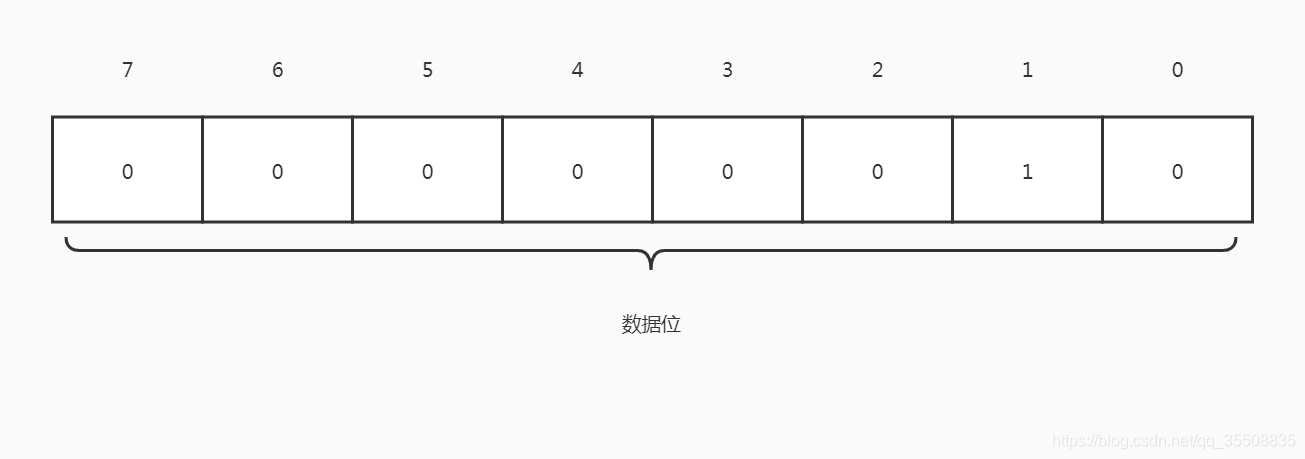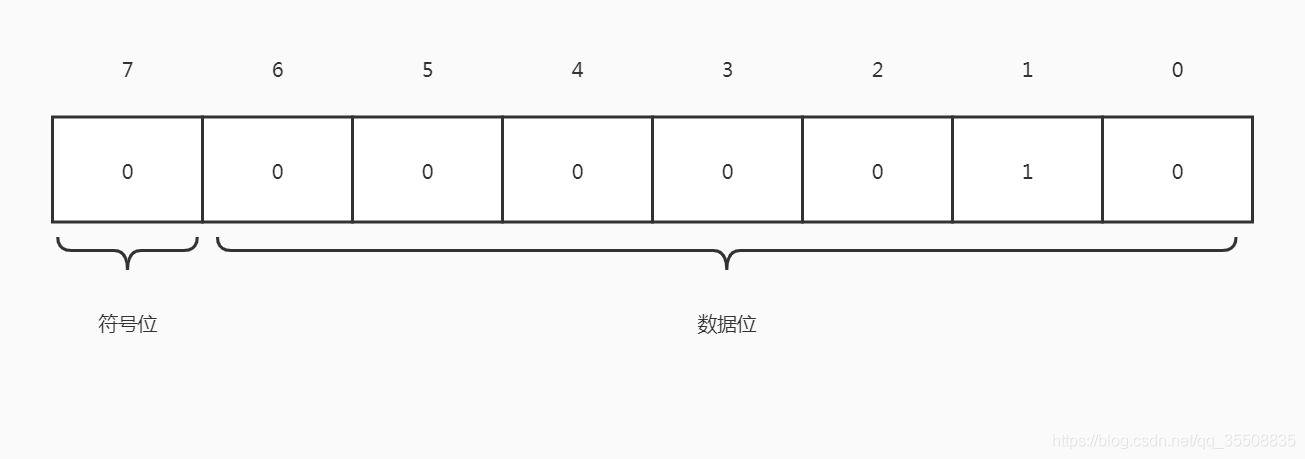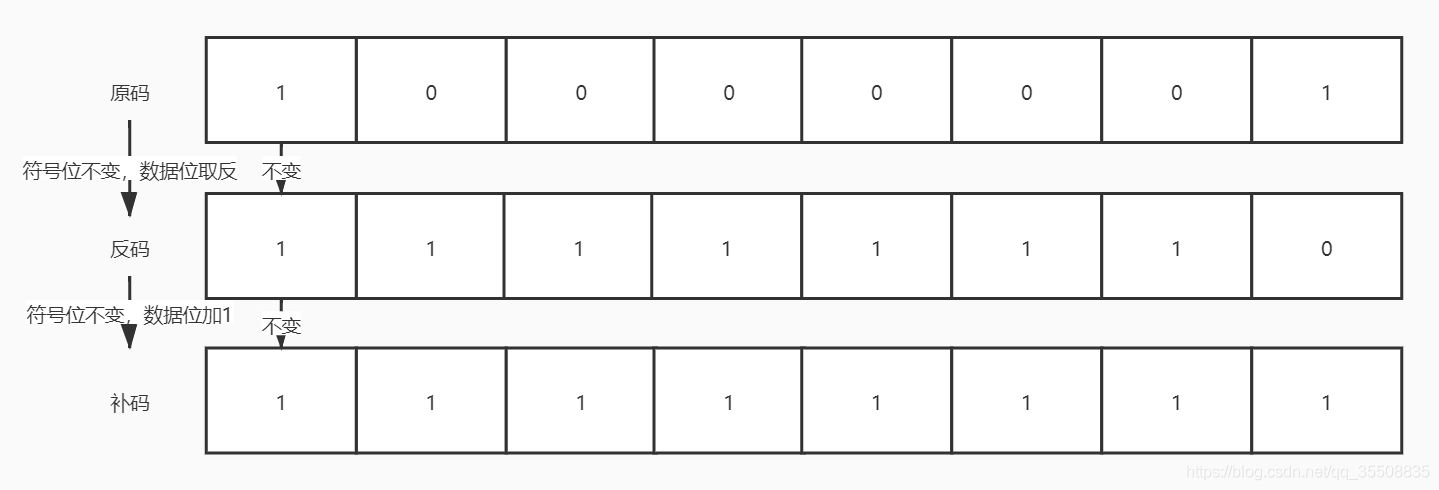### 你真的知道计算机是如何进行减法运算的吗？2 – 1

= 2 + (256 – 1)

= 2 + 255

= 257

= 257 – 256（减去溢出位）

= 1

0000 0010 – 0000 0001

= 0000 0010 + 1111 1111

= 1 0000 0001

= 0000 00012 – 1

= 2 + (256 – 1)

= 2 + 255

2 – 1

= 2 + (-1)

a、b皆为正整数，且a、b现有n种数值状态：

a – b = a + (-b)

a – b = a + (n – b)

a + (-b) = a + (n – b)

-b = n – b

-1 = 12 – 1

-1 = 11

a – 1 和a + 11都等于2

8位的数值共有256个状态
16位的数值共有65536个状态
32位的数值共有4294967296个状态

256 + (-1)

= 255

= 1111 1111

65536 + (-1)

= 65535

= 1111 1111 1111 1111

4294967296 + (-1)

= 4294967295

= 1111 1111 1111 1111 1111 1111 1111 1111

256 + (-2)

= 254

= 1111 1110

256 + (-8)

= 248

= 1111 10001000 0001

1111 1110

1111 1111

111 1111
– 000 0001

111 1111
– 000 0001

+000 0001

1000 0000
– 0000 0001

1000 0000
– 0000 0001

+ 1000 0000

1 0000 0000
– 0 0000 0001